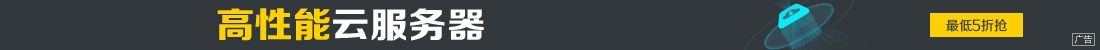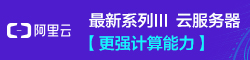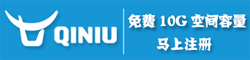# canvas学习之API整理笔记（二）

••```//获取canvas容器
var can = document.getElementById('canvas');
//创建一个画布
var ctx = can.getContext('2d');```

html代码：

`<canvas id="canvas" width="400" height="200" style="background:#fff;"></canvas>`

js代码：

```var canvas = document.getElementById('canvas');
var ctx = canvas.getContext('2d');
var ball = {  //小球属性，原点位置，速度，半径等。
x: 100,
y: 100,
vx: 4,
vy: 2,
color: 'blue',
draw: function() {
ctx.beginPath();
ctx.arc(this.x, this.y, this.radius, 0, Math.PI*2, true);
ctx.closePath();
ctx.fillStyle = this.color;
ctx.fill();
}
};
function draw() {
ctx.clearRect(0,0, canvas.width, canvas.height);  //绘制之前清除整个画布
ball.draw();  //在画布中绘制小球
ball.x += ball.vx;  //改变小球位置坐标
ball.y += ball.vy;  //改变小球位置坐标
if (ball.y + ball.vy > canvas.height-15 || ball.y + ball.vy < 15) {  //边界判断
ball.vy = -ball.vy;
}
if (ball.x + ball.vx > canvas.width-15 || ball.x + ball.vx < 15) {  //边界判断
ball.vx = -ball.vx;
}
window.requestAnimationFrame(draw);  //循环执行
}
draw();````ctx.clearRect(0,0, canvas.width, canvas.height);`

```ctx.fillStyle = 'rgba(255,255,255,0.3)';
ctx.fillRect(0, 0, canvas.width, canvas.height);```ImageData对象（由getImageData方法获取的）中存储着canvas对象真实的像素数据，它包含以下几个只读属性：

width

height

data

Uint8ClampedArray类型的一维数组，包含着RGBA格式的整型数据，范围在0至255之间（包括255）。简单讲，就是一个数组，每四个元素存储一个点的颜色信息，这四个元素分别对应为R、G、B、A的值（知道颜色取值的一眼就明白了，不知道的也没关系，后面有实例，一看就明白）。

`var myImageData = ctx.createImageData(width, height);`

`var myImageData = ctx.getImageData(left, top, width, height);`

html代码：

```<div id="color">hover处的颜色</div>
<canvas id="myCanvas" width="300" height="150"></canvas>```

js代码：

```var can = document.getElementById('myCanvas');
var ctx = can.getContext('2d');
var img = new Image();
img.src = "img_the_scream.jpg";
ctx.drawImage(img, 0, 0);
var color = document.getElementById('color');
function pick(event) {
var x = event.layerX;
var y = event.layerY;
var area = ctx.getImageData(x, y, 1, 1); //创建ImageData对象
var data = area.data;  //获取data属性（一个存储颜色rgba值的数组）
var rgba = 'rgba(' + data + ',' + data + ',' + data + ',' + data + ')';
color.style.color = rgba;
color.textContent = rgba;
}`img.src = "img_the_scream.jpg";`

`ctx.putImageData(myImageData, x, y);  //在画布的(x, y)点开始绘制myImageData所存储的像素信息。`

html代码：

`<canvas id="canvas" width="660" height="277"></canvas>`

```var canvas = document.getElementById('canvas');
var ctx = canvas.getContext('2d');
var img = new Image();
img.src = 'img_the_scream.jpg';
ctx.drawImage(img, 0, 0);
var imageData = ctx.getImageData(0,0,canvas.width, canvas.height); //获取ImageData
var colors = imageData.data; //获取像素信息
function invert() {
for (var i = 0; i < colors.length; i += 4) { //四个为一组
colors[i]   = 225 - colors[i];   // red
colors[i+1] = 225 - colors[i+1]; // green
colors[i+2] = 225 - colors[i+2]; // blue
colors[i+3] = 255;  //alpha
}
ctx.putImageData(imageData, 220, 0); //从(220, 0)开始绘制改变过的颜色
}
function toGray() {
for (var i = 0; i < colors.length; i += 4) {
var avg = (colors[i] + colors[i+1] + colors[i+2]) / 3;
colors[i] = avg; // red
colors[i+1] = avg; // green
colors[i+2] = avg; // blue
colors[i+3] = 255;  //alpha
}
ctx.putImageData(imageData, 440, 0); //从(440, 0)开始绘制改变过的颜色
}
invert();  //反转色
toGray();  //变灰色``````ctx.drawImage(myImage, 0.3, 0.5); //不提倡这样写，应该像下面这样处理
ctx.drawImage(myImage, Math.floor(0.3), Math.floor(0.5));```

```<canvas id="background-can" width="480" height="320"></canvas>
<canvas id="game-can" width="480" height="320"></canvas>```

• 将画布的函数调用集合到一起（例如，画一条折线，而不要画多条分开的直线）
• 使用不同的办法去清除画布(clearRect()、fillRect()、调整canvas大小)
• 有动画，请使用window.requestAnimationFrame() 而非window.setInterval()

OK，canvas常用的API就基本总结完了，靠这些API已经足够开发一些中型游戏了。比如之前自己写的实例demo之小游戏tinyHeart，就是用这些函数画出来的。关键是这些函数的组合使用，多多练习就好了。